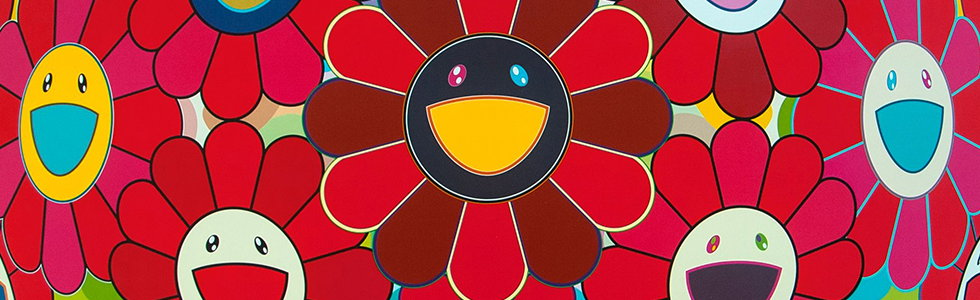# Takashi MurakamiCelestial Flowers
Flower BallINFINITY
SelectionDazzling Circus Embrace Peace and Darkness
SelectionFlowers of Hope
SelectionAn Homage to IKB 1957 From a Set of 3
SelectionKorin Tanquility
SelectionThe Golden Age: Korin - Kansei
SelectionAn Homage to Mono Pink 1960 From a Set of 3
SelectionAn Homage to Mono Gold 1960 From a Set of 3
SelectionWE ARE THE JOCULAR CLAN (3)
WE ARE THE JOCULAR CLANWE ARE THE JOCULAR CLAN (4)
WE ARE THE JOCULAR CLANWE ARE THE JOCULAR CLAN (5)
WE ARE THE JOCULAR CLANWE ARE THE JOCULAR CLAN (6)
WE ARE THE JOCULAR CLANWE ARE THE JOCULAR CLAN (7)
WE ARE THE JOCULAR CLANWE ARE THE JOCULAR CLAN (9)
WE ARE THE JOCULAR CLANWE ARE THE JOCULAR CLAN (10)
WE ARE THE JOCULAR CLANWE ARE THE JOCULAR CLAN (1)
WE ARE THE JOCULAR CLANWE ARE THE JOCULAR CLAN (2)
WE ARE THE JOCULAR CLANWE ARE THE JOCULAR CLAN (8)
WE ARE THE JOCULAR CLANWE ARE THE SQUARE JOCULAR CLAN (3)
WE ARE THE SQUARE JOCULAR CLANWE ARE THE SQUARE JOCULAR CLAN (2)
WE ARE THE SQUARE JOCULAR CLANWE ARE THE SQUARE JOCULAR CLAN (4)
WE ARE THE SQUARE JOCULAR CLANWE ARE THE SQUARE JOCULAR CLAN (5)
WE ARE THE SQUARE JOCULAR CLANWE ARE THE SQUARE JOCULAR CLAN (6)
WE ARE THE SQUARE JOCULAR CLANWE ARE THE SQUARE JOCULAR CLAN (7)
WE ARE THE SQUARE JOCULAR CLANWE ARE THE SQUARE JOCULAR CLAN (8)
WE ARE THE SQUARE JOCULAR CLANWE ARE THE SQUARE JOCULAR CLAN (9)
WE ARE THE SQUARE JOCULAR CLANWE ARE THE SQUARE JOCULAR CLAN (10)
WE ARE THE SQUARE JOCULAR CLANWE ARE THE SQUARE JOCULAR CLAN (1)
WE ARE THE SQUARE JOCULAR CLAN